Detalles de la revistaFormato
Revista
eISSN
2300-0929
Primera edición
19 Oct 2012
Calendario de la edición
4 veces al año
Idiomas
Inglés
Acceso abierto

# Determination of State Variables in Textile Composite with Membrane During Complex Heat and Moisture Transport

###### Páginas:328 - 334
Detalles de la revistaFormato
Revista
eISSN
2300-0929
Primera edición
19 Oct 2012
Calendario de la edición
4 veces al año
Idiomas
Inglés

The cotton-based composite is equipped with a single/double semipermeable membrane made of polyurethane (PU) (100%), which blocks liquid transport to the surrounding environment. The complex problem analyzed involves the coupled transport of water vapor within the textile material, transport of liquid water in capillaries, as well as heat transport with vapor and liquid water. The problem can be described using the mass transport equation for water vapor, heat transport equation, and mass transport equation for liquid moisture, accompanied by the set of corresponding boundary and initial conditions. State variables are determined using a complex multistage solution procedure within the selected points for each layer. The distributions of state variables are determined for different configurations of membranes.

#### Keywords

Introduction

Composites made of natural fibers are frequently subjected to a complex process of transport of heat and moisture. The problem is significant because of (i) the thermal comfort of the user in normal conditions and (ii) the adequacy of working conditions in clothing exposed to the heat flux of prescribed density. The semipermeable membrane made of polyurethane (PU) (100%) ensures the diffusion of water vapor to the surroundings, while simultaneously blocking the transport of liquid moisture outside (the sweat from the skin) and inside (the precipitation). The second membrane between the textile layers helps to secure the prescribed conditions in a cotton-based composite.

The effect of coupled transport on the protective performance of a garment has been investigated previously , with the model being developed using the balance concept and enthalpy formulation. The energy approach accompanied by adequate boundary conditions and Pennes’s approach of heat conduction in human skin have been presented by Song et al. . The problem can be also analyzed by means of the only state variable, viz., the temperature , and water vapor concentration .

The model of coupled heat and water vapor transport and the interaction with human comfort have been investigated by Li . Additional air layers are introduced inside the garment, which are determined by the active elements. A mathematical model that takes into account the water vapor sorption of fibers has been developed  to describe and predict the coupled heat and moisture transport in wool fabrics. The two-stage moisture sorption has been analyzed using the Fick’s diffusion and David–Nordon models. A mathematical simulation of the perception of thermal and moisture sensations also has been developed  using the physical mechanisms of heat and vapor transport, the neurophysiological responses, and the psychoneurophysiological relationships from experiments. On the basis of a mathematical model describing the coupled heat and moisture transfer in wool fabric, Haghi  has investigated the moisture sorption mechanisms in fabrics made from fibers with different degrees of hygroscopicity. For weakly hygroscopic fibers, such as polypropylene fiber, the moisture sorption can be described by a single Fickian diffusion with a constant diffusion coefficient.

Li et al.  have investigated the coupling mechanism of heat transfer and liquid moisture diffusion in porous textiles. An equation describing liquid diffusion is incorporated into the energy conservation equation and the mass conservation equations of water vapor and liquid moisture transfer, which include vapor diffusion, evaporation, and sorption of moisture by fibers. A dynamic model of liquid water transfer, coupled with moisture sorption, condensation, and heat transfer, in porous textiles has been developed by incorporating the physical mechanism of liquid diffusion in porous textiles . The same mathematical model of heat and mass transfer is coupled with the phase change of materials in porous textiles . The study of water vapor permeability of wet fabrics and the effect of air gaps between the skin and the fabric on the total relative cooling heat flow is analyzed by Hes and Araujo .

Puszkarz and Krucińska  investigate the comfort of double-layered knitted fabrics with applications addressed for specific users. Textiles with a comparable structure are tested for air permeability, and the results are compared with numerical calculations. Different aspects of textile materials in the microscale are analyzed by Grabowska and Ciesielska-Wróbel . The uncovered head causes significant heat and moisture loss from a newborn’s skin. The thickness of a composite textile bonnet that retains optimal skin parameters has also been analyzed . The study  presents the tests of heat insulation obtained for the newly developed garment compared with that of commercially available garments for babies, under different climatic conditions.

This paper is a continuation of previous investigations concerning the determination of state variables in complex composites [15, 17, 18, 19, 20, 21]. The main goal is to determine the distribution of state variables in the cotton-based composite subjected to complex heat, water vapor, and liquid water transport. The structure is equipped with a single membrane made of 100% PU (on the external boundary) and two membranes made of 100% PU (on the external boundary and between the textile layers), which can improve the user’s working conditions. The numerical simulation is always cheaper and gives more general results than the practical tests with measurable numerical and economic benefits.

The novel elements are the following. (i) Introduction of two cotton layers characterized by different material characteristics (cotton + Kevlar; acryl + cotton). (ii) Determination of state variables for cotton-based composite with single and double membranes to improve the thermal comfort and working conditions of the user.

Problem Formulation

Let us determine the problem of simultaneous transport of liquid moisture and water vapor within a capillary-porous textile material, combined with the heat transfer. The transport is unidirectional and is reduced to an optional cross section of the textile material determined within the 2D Cartesian coordinate system. The coordinate x = 0 denotes the lower part, whereas x = L represents the upper part of the material (Figure 1). The fibrous material is interleaved by capillaries, which transport the water vapor and liquid moisture (e.g., the sweat) from the skin to the surroundings. These capillaries are directed at a dominant angle of β.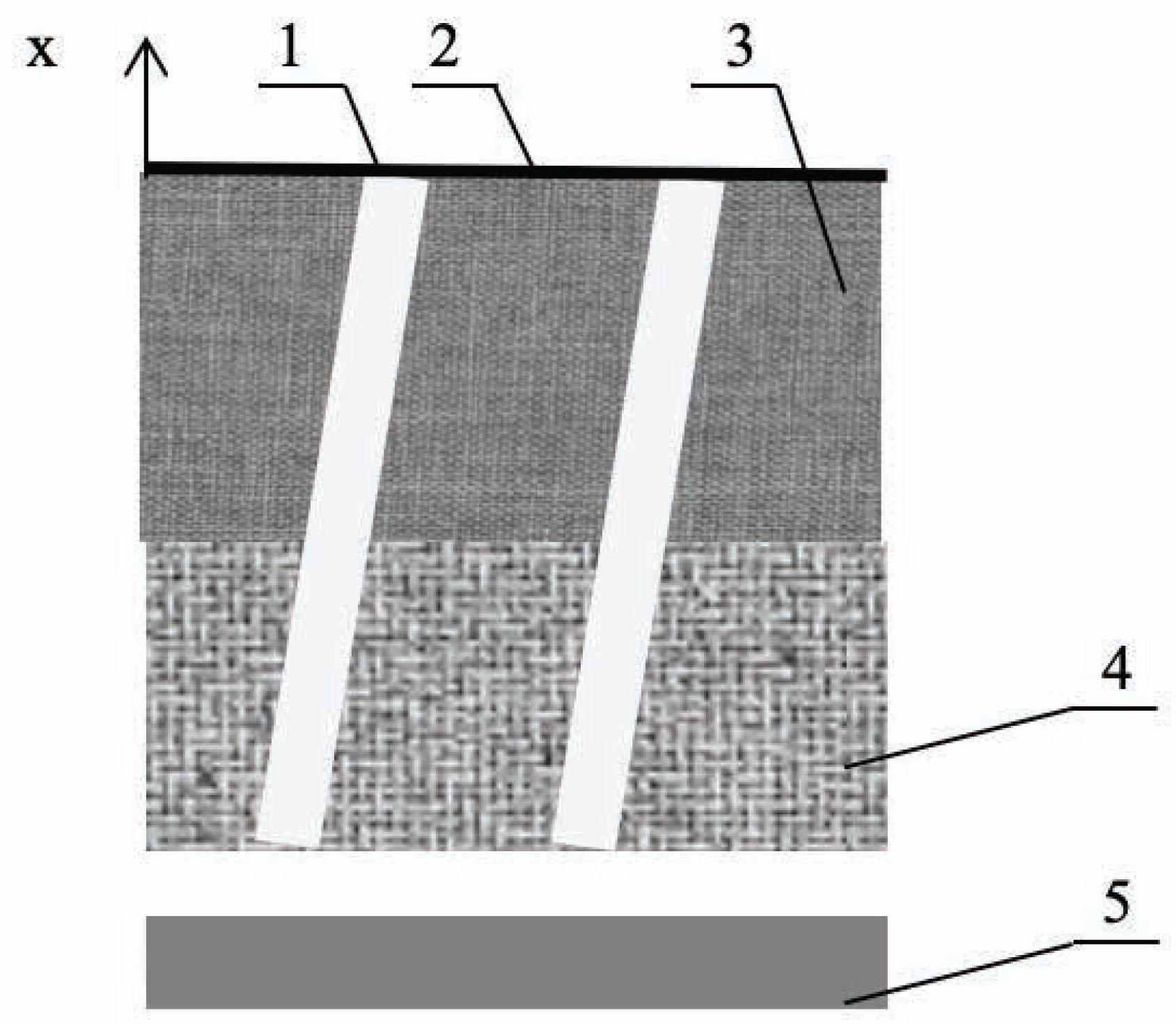Model of analyzed cotton-composite.1 – capillaries; 2 – membrane; 3 – external textile layer (acryl + cotton); 4 – internal textile layer (cotton + Kevlar); 5 – skin

The assumptions characterizing the physical model are the following [5, 6, 9, 10]: (i) Water vapor diffuses within the fibers and the interfiber void spaces. Liquid is transported by surface tension to the regions of lesser concentrations. Heat is transported by conduction within fibers and by convection from the outer surfaces to the void spaces as well as within these spaces. (ii) The volume increase caused by mass diffusion and liquid transport can be neglected. (iii) Orientation of the fibers can be significant, although the diameters are small, and water vapor is transported through the interfiber spaces faster than within the fibers. The internal structure is different in woven fabrics, knitted fabrics, and nonwovens, and it should be always deeply analyzed. (iv) Though the combined transport is a disequilibrium process, instantaneous thermodynamic equilibrium can be introduced between the textile material and the fluid in the free spaces and capillaries irrespective of time characteristics. Textile fibers have small diameters and large surface/volume ratio. (v) The inertial force is neglected considering the low velocities. (vi) The air/vapor mixture is at saturation point in the presence of the liquid phase. (vii) Capillaries are assumed to be of the same diameter and ensure the continuous flow, i.e., the liquid transport is assumed to satisfy the cumulative frequency and linear distribution.

Introducing the balance concept, we can determine the following: (i) the mass transport equation for water vapor – Eq. (1); (ii) the heat transport equation – Eq. (2); (iii) the mass transport equation for liquid moisture – Eq. (3). $∂(waεa)∂t+w1∂(wfεf)∂x−εahmSv(w*−wa)=−1ξa∂∂x[Da∂(waεa)∂x]$ {{\partial \left( {{w_a}{\varepsilon _a}} \right)} \over {\partial t}} + {w_1}{{\partial \left( {{w_f}{\varepsilon _f}} \right)} \over {\partial x}} - {\varepsilon _a}{h_m}{S_v}\left( {{w^*} - {w_a}} \right) = - {1 \over {{\xi _a}}}{\partial \over {\partial x}}\left[ {{D_a}{{\partial \left( {{w_a}{\varepsilon _a}} \right)} \over {\partial x}}} \right] $ρc∂T∂t−w1λ1∂(wfεf)∂t−w2λ1∂(wfεf)∂t+εaλhmSv(w*−wa)=−∂∂x[A∂T∂x]$ \rho c{{\partial T} \over {\partial t}} - {w_1}{\lambda _1}{{\partial \left( {{w_f}{\varepsilon _f}} \right)} \over {\partial t}} - {w_2}{\lambda _1}{{\partial \left( {{w_f}{\varepsilon _f}} \right)} \over {\partial t}} + {\varepsilon _a}\lambda {h_m}{S_v}\left( {{w^*} - {w_a}} \right) = - {\partial \over {\partial x}}\left[ {A{{\partial T} \over {\partial x}}} \right] $∂(ρlεl)∂t+w2∂(wfεf)∂t+εahmSv(w*−wa)=−1ξl∂∂x[Dl∂(ρlεl)∂x]$ {{\partial \left( {{\rho _l}{\varepsilon _l}} \right)} \over {\partial t}} + {w_2}{{\partial \left( {{w_f}{\varepsilon _f}} \right)} \over {\partial t}} + {\varepsilon _a}{h_m}{S_v}\left( {{w^*} - {w_a}} \right) = - {1 \over {{\xi _l}}}{\partial \over {\partial x}}\left[ {{D_l}{{\partial \left( {{\rho _l}{\varepsilon _l}} \right)} \over {\partial x}}} \right] where $w1=εaεa+εl$ {w_1} = {{{\varepsilon _a}} \over {{\varepsilon _a} + {\varepsilon _l}}} ; $w2=εlεa+εl$ {w_2} = {{{\varepsilon _l}} \over {{\varepsilon _a} + {\varepsilon _l}}} ; w1 + w2 = 1.

The mass transfer equation, i.e., Eq. (1), determines the transport of water vapor as follows: (i) within the void spaces between the fibers in time – the first term on the left-hand side; (ii) in the fibers from the skin to the surroundings – the second term; (iii) from the current concentration to the saturation conditions that cause vapor condensation – the third term. The heat transport equation, i.e., Eq. (2), describes the following: (i) the heat transported with liquid water – the first term on the left-hand side; (ii) the heat transported during sorption/desorption of water vapor and liquid moisture in fibers – the second and third terms; (iii) the latent heat of condensation – the fourth term. The mass transport equation for liquid, i.e., Eq. (3), defines the transport of moisture as follows: (i) along the capillaries in time – the first term on the left-hand side; (ii) in the fibers with respect to time – the second term; (iii) the current transport of liquid water to attain the saturation condensation – the third term.

The sum of the volume fractions is represented as follows. $εa+εl+εf=1⇒εa+εl=1−εf$ {\varepsilon _a} + {\varepsilon _l} + {\varepsilon _f} = 1 \Rightarrow {\varepsilon _a} + {\varepsilon _l} = 1 - {\varepsilon _f}

The sorption/desorption of water vapor between the fibers and the interfiber spaces is a complex process [5, 6]. The first stage of sorption is described by Fick’s law and the constant diffusion coefficient of the material. The sorption/desorption process within some materials possessing strongly hygroscopic properties (cf., wool) is determined by Fick’s law during the entire process [5, 6, 9, 10]. Assuming a short duration of time, the problem is described by the first phase of sorption. The discrete relations have the following form. $dwfdt=R1 for WC<0.185 and tteq$ \matrix{ {{{d{w_f}} \over {dt}} = {R_1}\,{\rm{for}}\,{W_C} < 0.185\,\,\,{\rm{and}}\,t < {t_{eq}};} \hfill \cr {{{d{w_f}} \over {dt}} = {1 \over 2}{R_1} + {1 \over 2}{R_2}\,\,\,{\rm{for}}\,{W_C} \ge 0.185} \,\,\,{\rm{and}}\,t < {t_{eq}};\hfill \cr {{{d{w_f}} \over {dt}} = {R_{2}}\,\,{\rm{for}}\,t > {t_{eq}}} \hfill} $WC=wf(x,Rf,t)ρf$ {W_C} = {{{w_f}\left( {x,{R_f},t} \right)} \over {{\rho _f}}}

The equilibrium time, teq, is determined experimentally, which depends on the textile material and is in the range of 540–600 s [5, 6, 8]. For weakly hygroscopic fibers such as polypropylene, the moisture sorption is described by a single Fickian diffusion with a constant diffusion coefficient. The sorption rate during the first stage, R1, is defined by the water vapor transport in dry cylindrical fibers [9, 10] according to Fick’s diffusion. $dwfdt=R1(x,t)=1Rf∂∂Rf[RfDa(x,t)∂wf(x,Rf,t)∂Rf]$ {{d{w_f}} \over {dt}} = {R_1}\left( {x,t} \right) = {1 \over {{R_f}}}{\partial \over {\partial {R_f}}}\left[ {{R_f}{D_a}\left( {x,t} \right){{\partial {w_f}\left( {x,{R_f},t} \right)} \over {\partial {R_f}}}} \right]

The boundary conditions at the skin (x = 0) determine the concentration of saturated water vapor, the specified temperature, and the constant volume fraction of fibrous material in the following form. $wa(0,t)=w*;T(0,t)=T0;εf(0,t)=const$ {w_a}\left( {0,t} \right) = {w^*};T\left( {0,t} \right) = {T_0};{\varepsilon _f}\left( {0,t} \right) = {\rm{const}}

Let us assume that the external boundary x = L is unprotected against disadvantageous working conditions. It is subjected to water vapor convection from the void spaces, as shown in Eq. (9). Heat is transferred by radiation, convection, and latent heat of evaporation, as in Eq. (10). Liquid moisture is transferred by convection, shown in Eq. (11). The appropriate conditions are expressed below. $Da∂[wa(L,t)εa(L,t)]∂x=εaεa+εlhw(wa−w∞)$ {D_a}{{\partial \left[ {{w_a}\left( {L,t} \right){\varepsilon _a}\left( {L,t} \right)} \right]} \over {\partial x}} = {{{\varepsilon _a}} \over {{\varepsilon _a} + {\varepsilon _l}}}{h_w}\left( {{w_a} - {w_\infty }} \right) $A∂T(L,t)∂x=σT4+h(T−T∞)+εlεa+εlhmλ(w*−w∞)$ A{{\partial T\left( {L,t} \right)} \over {\partial x}} = \sigma {T^4} + h\left( {T - {T_\infty }} \right) + {{{\varepsilon _l}} \over {{\varepsilon _a} + {\varepsilon _l}}}{h_m}\lambda \left( {{w^*} - {w_\infty }} \right) $Dl∂[ρl(L,t)εl(L,t)]∂x=εlεa+εlhm(w*−w∞)$ {D_l}{{\partial \left[ {{\rho _l}\left( {L,t} \right){\varepsilon _l}\left( {L,t} \right)} \right]} \over {\partial x}} = {{{\varepsilon _l}} \over {{\varepsilon _a} + {\varepsilon _l}}}{h_m}\left( {{w^*} - {w_\infty }} \right)

The external boundary can be alternatively protected by the semipermeable membrane. The membrane ensures the partial convection of water vapor from the void spaces by the reduced mass convection coefficient hwe, represented by Eq. (12). Heat is transported to the surroundings by radiation and convection [Eq. (13)]. Liquid is not transported to the surroundings because the pore dimensions prevent drop-wise penetration, as shown in Eq. (14). $Da∂[wa(L,t)εa(L,t)]∂x=εaεa+εlhwe(wa−w∞)$ {D_a}{{\partial \left[ {{w_a}\left( {L,t} \right){\varepsilon _a}\left( {L,t} \right)} \right]} \over {\partial x}} = {{{\varepsilon _a}} \over {{\varepsilon _a} + {\varepsilon _l}}}{h_{we}}\left( {{w_a} - {w_\infty }} \right) $A∂T(L,t)∂x=σT4+he(T−T∞)$ A{{\partial T\left( {L,t} \right)} \over {\partial x}} = \sigma {T^4} + {h_e}\left( {T - {T_\infty }} \right) $Dl∂[ρl(L,t)εl(L,t)]∂x=0$ {D_l}{{\partial \left[ {{\rho _l}\left( {L,t} \right){\varepsilon _l}\left( {L,t} \right)} \right]} \over {\partial x}} = 0

Let us next introduce the two-layered textile structure. The internal layer is made of cotton (80%) and Kevlar (20%) of thickness 7 × 10−3 m. The external layer is made of acrylic fiber (80%) and cotton (20%) of thickness 8 × 10−3 m. The surface mass is equal to 0.500 kg/m2 for Kevlar, 0.300 kg/m2 for cotton, and 0.350 kg/m2 for acrylic fiber. The working time in the test clothing is limited and does not exceed teq = 540 s; the diffusion in the fibers is determined by the first stage of sorption.

The material shows unidirectional transport of moisture and heat. The diffusion coefficients within the fibers are assumed according to previous reports . $cotton:Da fiber=[0.8481+50.6wfρ−1100(wfρ)2].10−14;acryl:Da fiber=[1.1200−410wfρ−8200(wfρ)2].10−13;$ \matrix{ {{\rm{cotton}}:{D_{a\,fiber}} = \left[ {0.8481 + 50.6{{{w_f}} \over \rho } - 1100{{\left( {{{{w_f}} \over \rho }} \right)}^2}} \right]{{.10}^{ - 14}};} \hfill \cr {{\rm{acryl}}:{D_{a\,fiber}} = \left[ {1.1200 - 410{{{w_f}} \over \rho } - 8200{{\left( {{{{w_f}} \over \rho }} \right)}^2}} \right]{{.10}^{ - 13}};} \hfill}

Kevlar Da fiber = 1.4e−13.

The diffusion coefficient of water vapor in the air is Da = 2.5e−5. The “internal” material porosities within the fibers are constant and are equal to the following values: $Kevlar+cotton:ε=0.350; acryl+cotton: ε=0.200$ {\rm{Kevlar}} + {\rm{cotton}}:\varepsilon = 0.350;\,\rm{acryl} + \rm{cotton}:\,\,\,\varepsilon = 0.200

Of course, the global value of the diffusion coefficient within the fibers is determined using any homogenization method, e.g., the simplest “rule of mixture”.

The heat transport coefficients in textile fibers have the following values : $cotton:Afiber=[44.10+63.00wfρ].10−3acryl:Afiber=28.80×10−3;Kevlar:Afiber=51.80×10−3.$ \matrix{ {{\rm{cotton}}:{A_{fiber}} = \left[ {44.10 + 63.00{{{w_f}} \over \rho }} \right]{{.10}^{ - 3}}} \hfill \cr {{\rm{acryl}}:{A_{fiber}} = 28.80 \times {{10}^{ - 3}};\,{\rm{Kevlar}}:{A_{fiber}} = 51.80 \times {{10}^{ - 3}}.} \hfill}

The heat of sorption of water vapor in the fibers, λv, determines the part of heat transported with moisture during sorption on the external surface of the fibers. The volumetric heat capacity c is the heat transferred to increase the temperature. $cotton:λvfiber=1030.9 exp[−22.30wfρ]+2522.00acryl:λvfibrer=2522.00; Kevlar:λvfiber=2522.00cotton:cfiber=(1663.00+4184.00wfρ)[1610.90(1+wfρ)]−1acryl:cfiber=1610.90;Kevlar:cfiber=1715.00$ \matrix{ {{\rm{cotton}}:{\lambda _{vfiber}} = 1030.9\,\exp \left[ { - 22.30{{{w_f}} \over \rho }} \right] + 2522.00} \hfill \cr {{\rm{acryl}}:{\lambda _{vfibrer}} = 2522.00;\,{\rm{Kevlar}}:{\lambda _{vfiber}} = 2522.00} \hfill \cr {{\rm{cotton}}:{c_{fiber}} = \left( {1663.00 + 4184.00{{{w_f}} \over \rho }} \right){{\left[ {1610.90\left( {1 + {{{w_f}} \over \rho }} \right)} \right]}^{ - 1}}} \hfill \cr {{\rm{acryl}}:{c_{fiber}} = 1610.90;{\rm{Kevlar}}:{c_{fiber}} = 1715.00} \hfill}

The volume fraction of the fibrous material is equal to ef = 0.80 for the internal layer and ef = 0.85 for the external layer. The initial parameters are the same for the structure and the surroundings: T0 = T = 21°C; w0 = w = 0.65. The composite is subjected at t = 0 to the following conditions at the skin: T = 19°C; w = 1.0.

The radii of monofilaments are assumed as follows: cotton Rf = 0.9 × 10−5 m; acryl Rf = 10−5 m; and Kevlar Rf = 10−5 m. The corresponding fiber densities are as follows: cotton r = 1.35 × 103 m; acryl r = 1.40 × 103 m; and Kevlar r = 1.45 × 103 m.

The heat and mass transport coefficients from the external surface are assumed as follows: h = 8 W/(m2·K); he = 4 W/(m2·K); hw = 0.2 m/s; hwe = 0.05 m/s; the mass transport coefficient within the fabric is hm = 0.015 m/s. For simplicity, let us assume that Dl = Da and λl = λa. The effective tortuosity of the fabric by diffusion of water vapor and liquid phase is ξl = ξa = 1.2; the effective porosity of the fabric is ɛ = 0.8. The latent heat of evaporation of water λ= is 2256 kJ/kg.

Numerical Solution

The problem is solved numerically using an iterative procedure of the following operational sequence.

Water vapor concentration within the fibers wf is determined from Eq. (4), i.e., Fick’s diffusion within a fibrous material.

Two-factor formula (ɛawa) is calculated from the mass transfer equation for water vapor, i.e., Eq. (1).

Volume fraction of the liquid phase ɛl can be determined from the mass transport equation for liquid moisture, i.e., Eq. (3).

Volume fraction of water vapor ɛa is computed from the sum of the volume fractions, viz., Eq. (4).

Water vapor concentration in the air filling the interfiber void space wa is consequently defined by a simple division: (ɛawa)/ɛa.

Temperature T is calculated from the heat transport equation, i.e., Eq. (2).

The textile structure with material characteristics as in Eq. (18) is exposed to coupled transport for 400 s; the problem can be solved numerically from t = 0 to the final value t = 400 s with a time step equal to 25 s.

Let us first analyze the textile structure made of two textile layers without any membrane. The location of the calculation points is shown in Figure 2a. The distributions of the vapor concentrations in the air filling the interfiber void space wa versus time are depicted by Figure 2b. The concentration wa increases rapidly with time to the maximal value irrespective of the calculation point. Next, the concentrations decrease slightly (3–3.5%) to a constant level. The vapor concentrations at the points located further toward the external boundary are reduced in relation to the values at the points located closer. The reason is convection of both vapor and fluid from the external surface to the surroundings.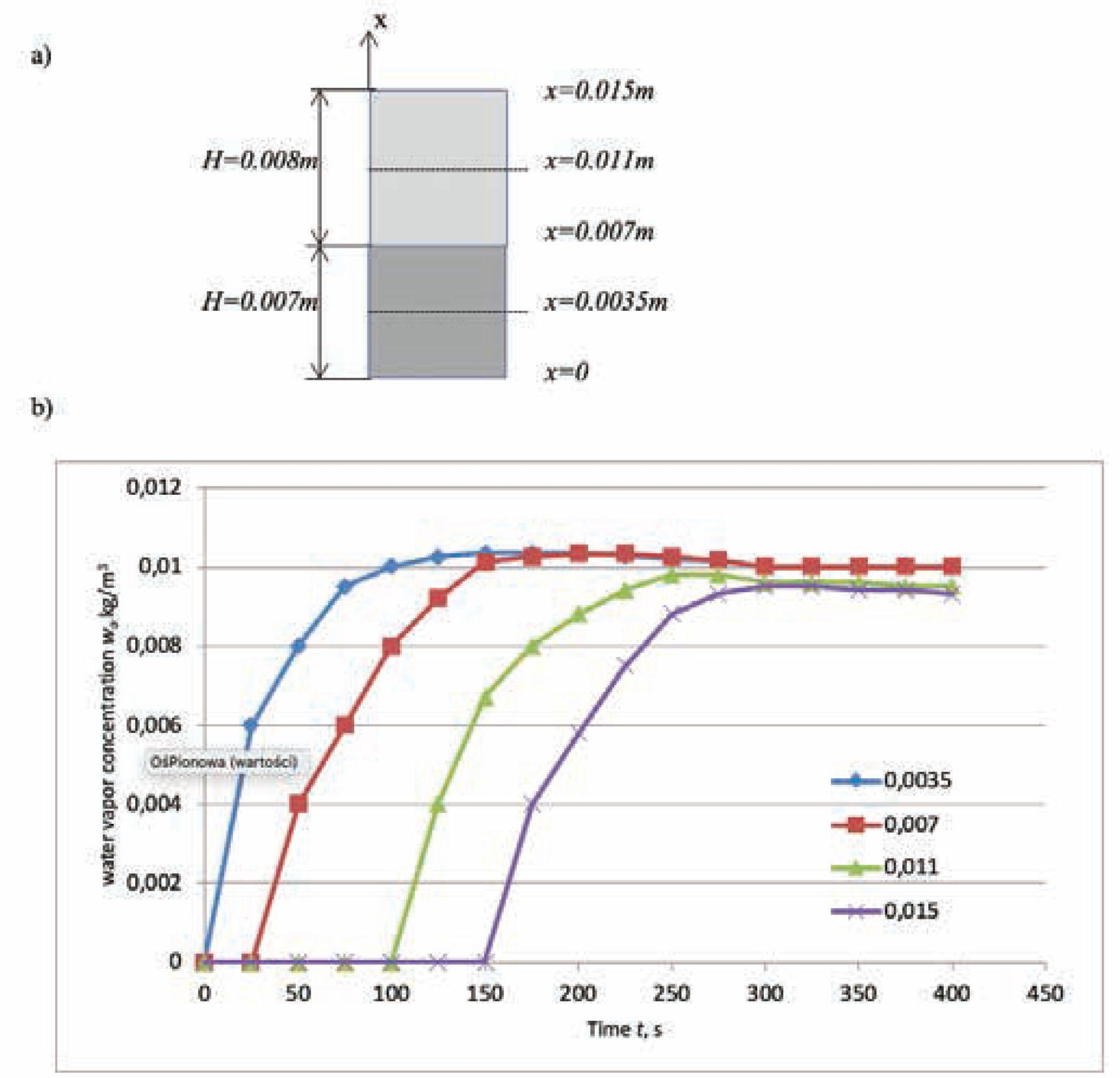a) Location of calculation points; b) Water vapor concentration in air filling interfiber void space wa versus time for different coordinates x of simple cotton-composite

The water vapor concentration within the fibers wf versus time is shown in Figure 3. The curves increase fast in time and reach concentration values close to maximum at time t = 200–250 s, and then, the values increase insignificantly to the maximal value at the final time point. The water vapor concentration depends on the location within the material. The values within the points x = 0.0035 m and x = 0.007 m are considerably greater than the values at other points. Different courses are seen for various materials (cotton and Kevlar; acryl + cotton), which show different characteristics of water vapor penetration.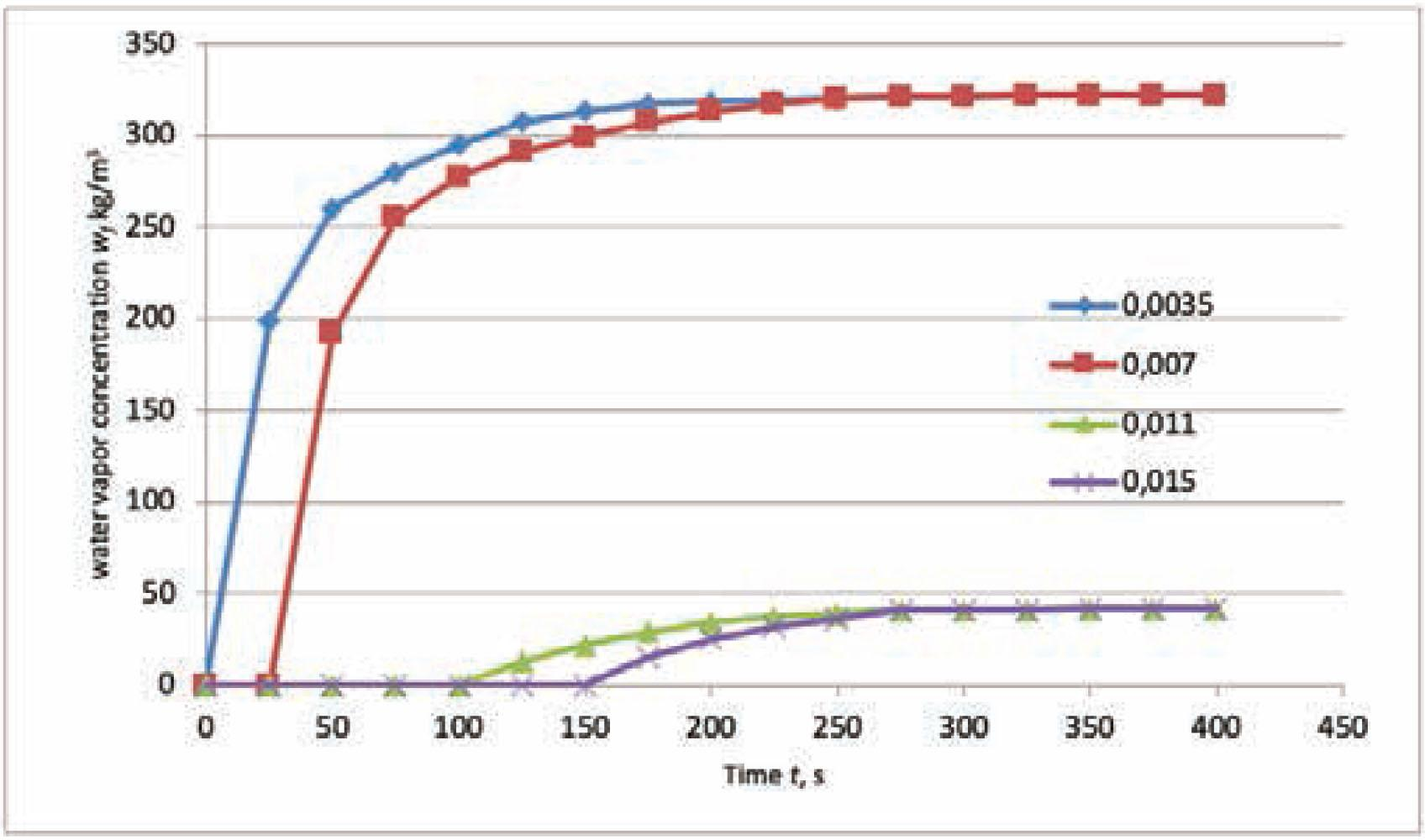Water vapor concentration within fibers wf versus time for different coordinates x of simple cotton-composite

The course of temperature versus time is shown in Figure 4. The temperature at the calculation points situated closer to the skin is higher, and the courses are different depending on the location. The points located closer to the skin are characterized by a rapidly reduced course at the beginning of the calculation time. In contrast, the temperature at the points located further from the skin decreases softly at the beginning of the test period. Next, the temperature reduction is substantial. The maximal difference in temperature at the end of the time range is 0.3°C (about 1.8%). The temperatures for x = 0.0035 m and x = 0.007 m are reduced in relation to the other two values.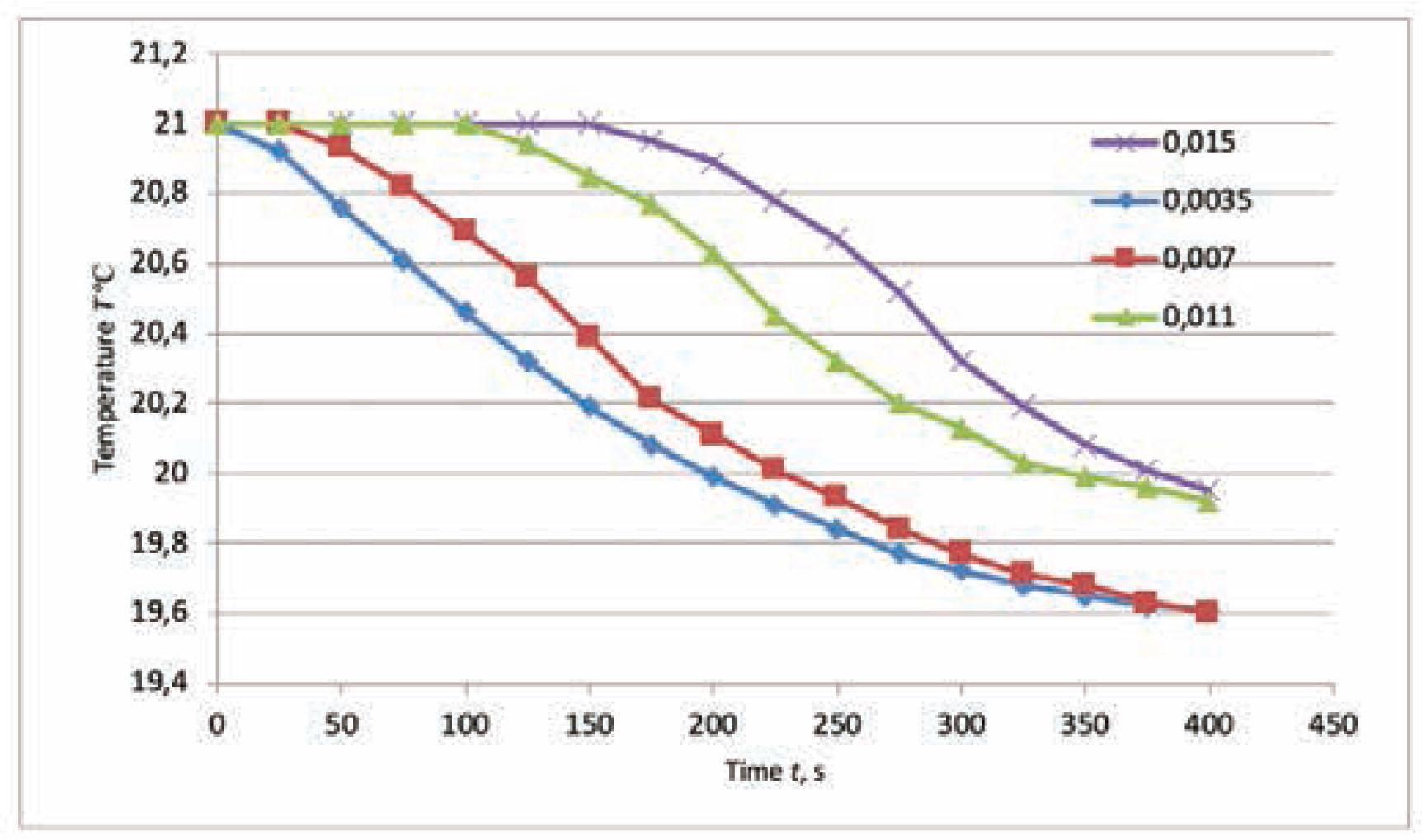Temperature T versus time for different coordinates x of simple cotton-composite

Generally speaking, the computed distributions of the state variables are concomitant to those obtained by other authors [9, 10].

The external boundary can be also protected by a semipermeable membrane made of polymer/polypropylene of thickness 0.25 × 10−3 m. The water vapor is now transported to the surroundings, whereas the liquid water outside and inside is blocked because of the pore dimensions. The layers are made of the same materials (cotton + Kevlar, acryl + cotton). The solution is typical for personal protective equipment, firefighter clothing, and so on. The water vapor concentration in the air filling the interfiber space (wa) versus time is depicted in Figure 5. The courses in Figures 2b and 5 are similar in the first part of the time range. The final concentrations are different, as shown in Figure 2b, irrespective of time and location. It is caused by the membrane, which blocks the transport of vapor to the surroundings and equalizes the values irrespective of location point. The curves are asymptotic, but the maximal concentrations are reached at different times, which in turn depend on the location of the point, i.e., from t = 150 s for x = 0.0035 m to t = 325 s for x = 0.015 m.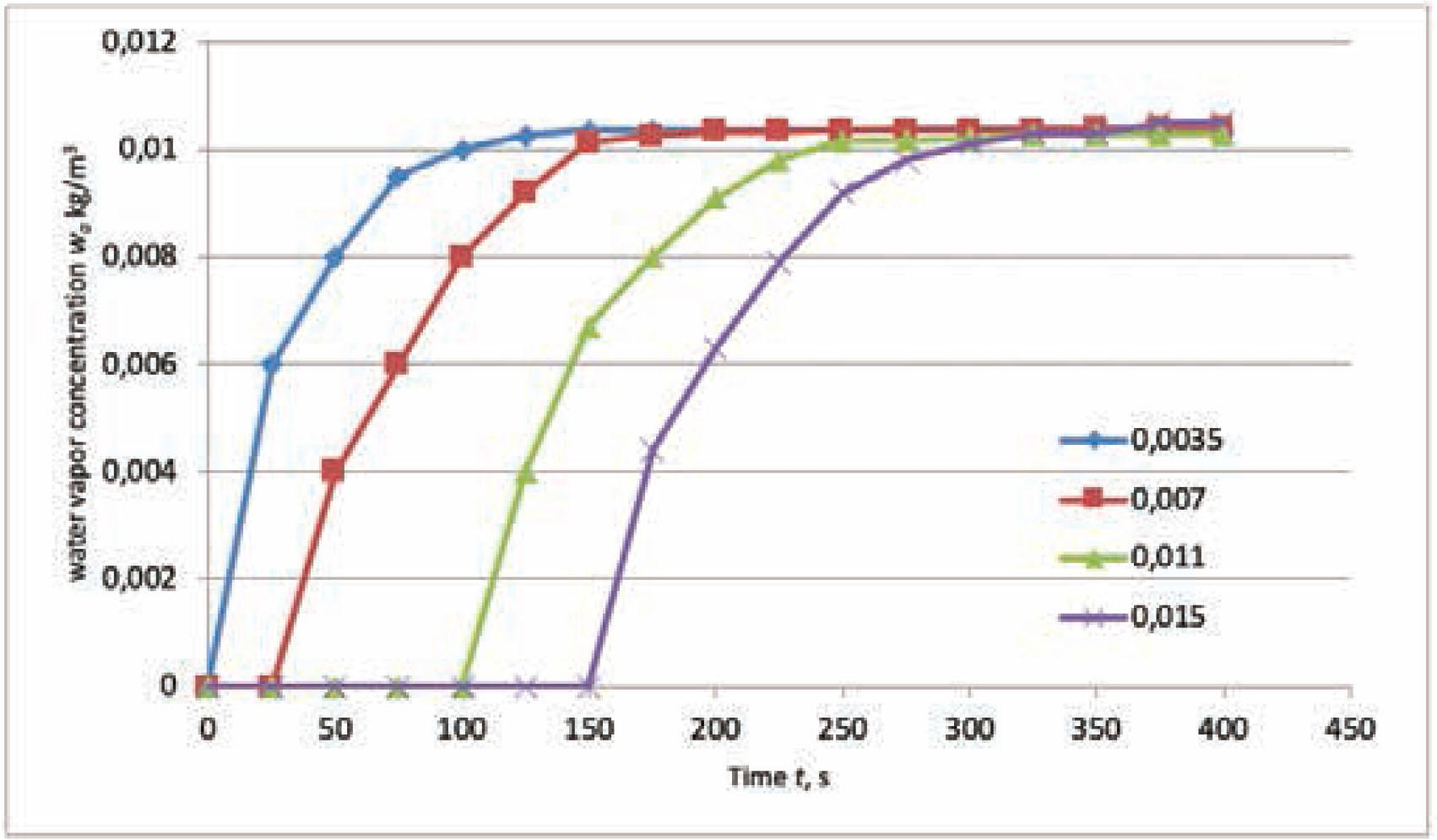Water vapor concentration in air filling interfiber void space wa versus time for different coordinates x of cotton-composite with single membrane

The water vapor concentrations within the fibers, wf, are depicted in Figure 6. The membrane on the external surface of the cotton-based composite protects against vapor loss to the surroundings. Therefore, the values of vapor concentrations in the fibers increase continually irrespective of the time to the saturation value at the maximal time. The difference in the values at different calculation points is caused by the diverse nature of the materials in the layers. The course of temperature versus time is similar to that shown in Figure 4.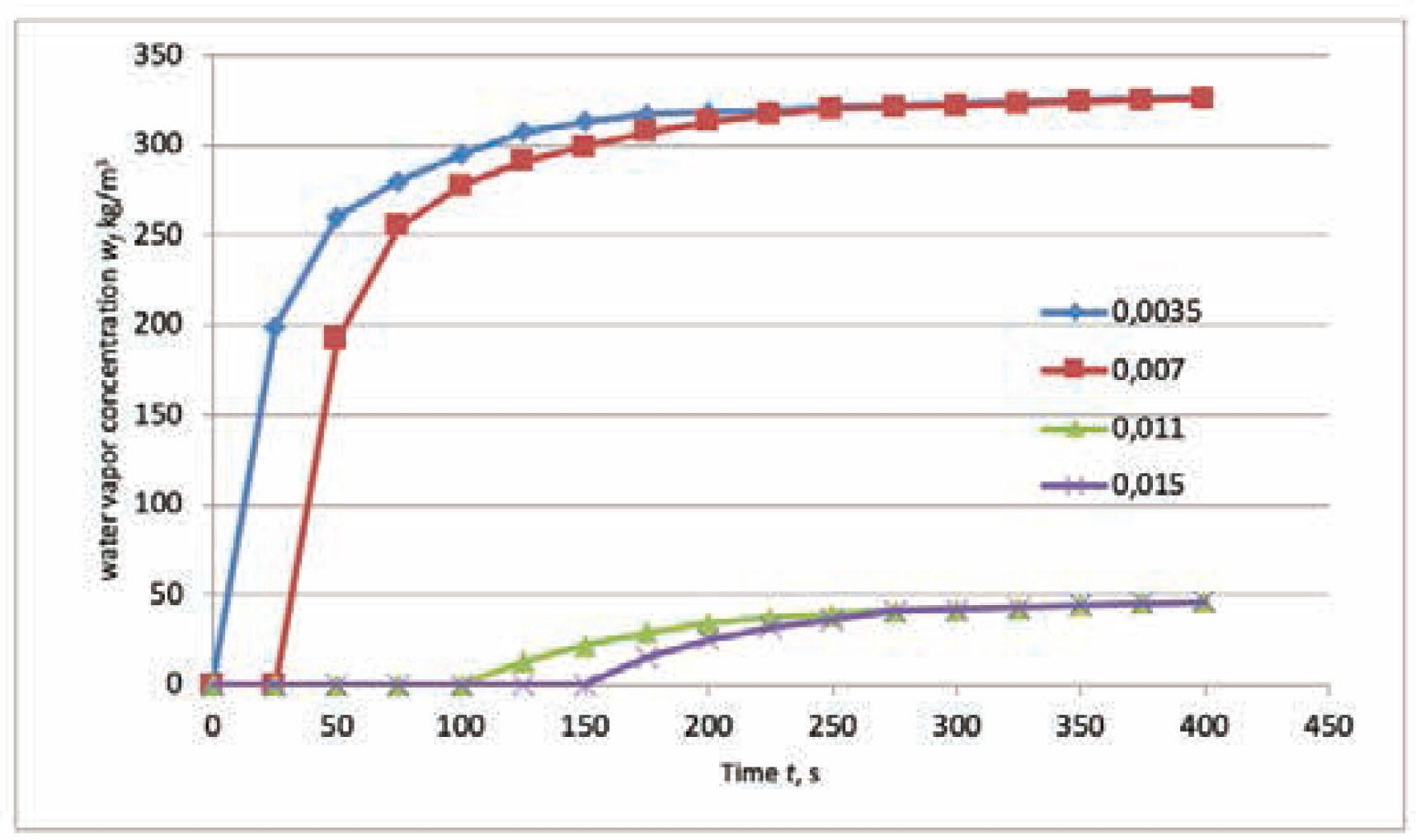Water vapor concentration within fibers wf versus time for different coordinates x of cotton-composite with single membrane

The next composite is equipped with two membranes, the first is between two cotton layers, and the second is on the external boundary. The structure can be implemented into the personal protective equipment for firemen subjected to thermal radiation from the fire source. The vapor transport to the surroundings is blocked, which results in an increased level of water within the internal layer and a constant skin temperature due to contact with the coolant. The water vapor concentration within the interfiber space, wa, versus time is shown in Figure 7. The initial values of vapor concentrations increase rapidly for x = 0.0035 m and x = 0.007 m; the courses are similar to the corresponding curves in Figure 3. The values at the other points increase softly, which is caused by the internal membrane between the textile layers. The external membrane yields the maxima of concentrations at the final time point, and these values are comparable within all calculation points.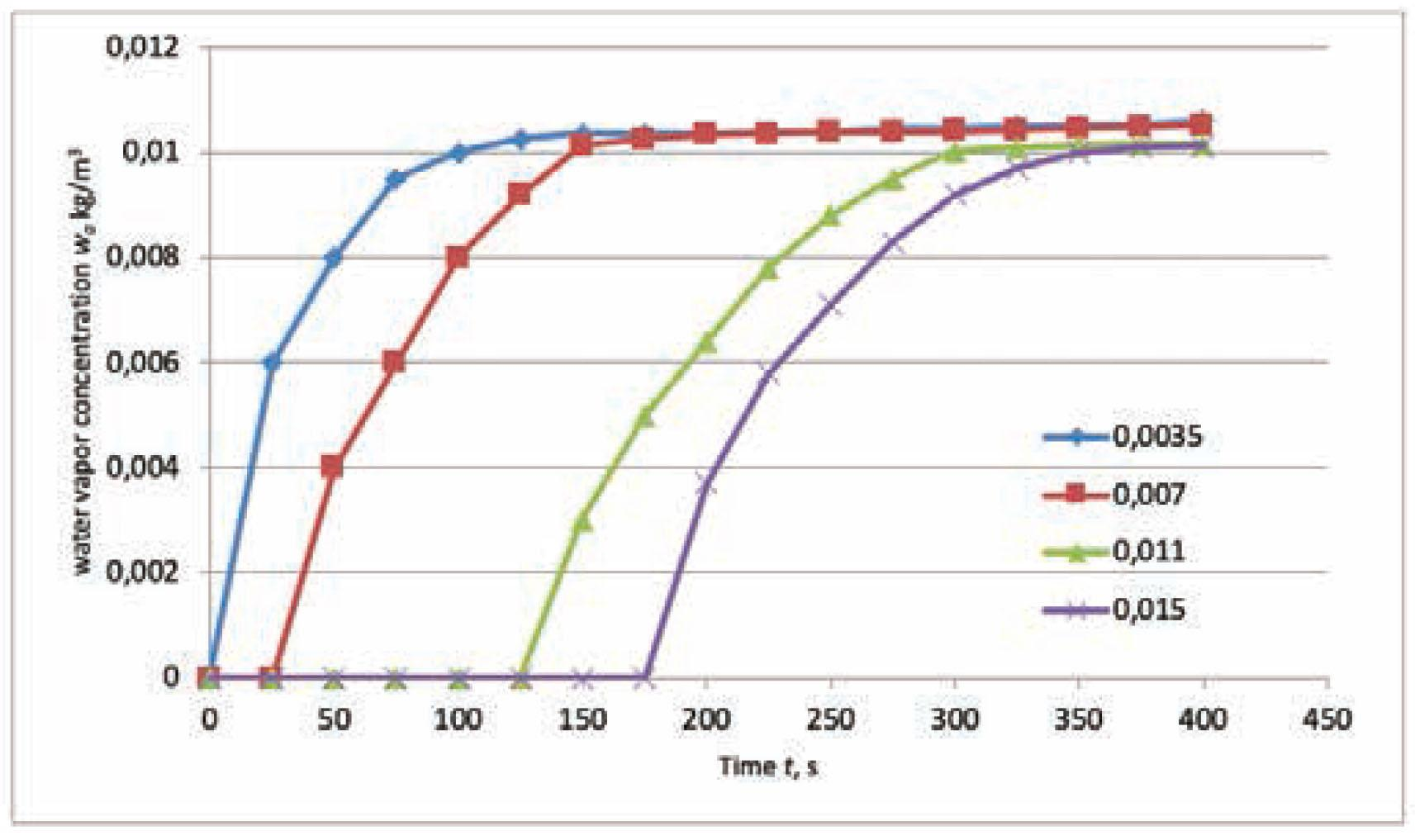Water vapor concentration in air filling interfiber void space wa versus time for different coordinates x of cotton-composite with double membrane
Conclusions

The combined transport of water vapor, liquid moisture, and heat in cotton-based composite is a complex phenomenon. The physical model introduces different simplifications, e.g., only the dominant angle of the pore axes, only Fick’s diffusion, and so on. However, the model proposed is complicated, although the approximated solution is obtained in a few consecutive steps. The obtained distribution of state variables for a simple structure without membranes is concomitant with that published by other authors [9, 10].

The light protective clothing for firefighters does not contact the flame and withstands a short exposure time to heat flux of prescribed density. The membrane is the additional barrier protecting against complex heat, moisture, and liquid transport, as well as retaining an adequate temperature on the skin. The phenomenon is combined because heat is transported with liquid water, by sorption/desorption of water vapor and liquid moisture in the fibers, and by the phase change (the latent heat of condensation). The textile layers are made of cotton with Kevlar/acryl, which improve both the moisture transport from the skin and the personal comfort of user. The single- and double-membrane structure causes an increase in water vapor concentrations within the void spaces wa in time. Additionally, the courses of the maximal values for samples with membrane are more convergent than the courses of samples without membrane.

The solution of the complex transport process is determinable by significant simplifications of the model. The problem is affected by the optimization balance, i.e., the most accurate results versus the most beneficial computational effort. Thus, the analysis can be developed to find the simplest possible solution, for instance, by some additional simplifications of the model. The problem can be also applied to optimize the shape and material characteristics of textile layers within the composite. The finishing procedure and its influence on the material characteristics can be additionally discussed .

Chitrphiromsri, P., Kuznetsov, A. V. (2005). Modeling heat and moisture transport in firefighter protective clothing during flash fire exposure. Heat and Mass Transfer, 41, 206–215. ChitrphiromsriP. KuznetsovA. V. 2005 Modeling heat and moisture transport in firefighter protective clothing during flash fire exposure Heat and Mass Transfer 41 206 215 10.1007/s00231-004-0504-x Search in Google Scholar

Song, G., Barker, R. L., Hamouda, H., Kuznetsov, A. V., Chitrphiromsri, P., et al. (2004). Modeling the thermal protective performance of heat resistant garments in flash fire exposure, Textile Research Journal, 74(12), 1033–1040. SongG. BarkerR. L. HamoudaH. KuznetsovA. V. ChitrphiromsriP. 2004 Modeling the thermal protective performance of heat resistant garments in flash fire exposure Textile Research Journal 74 12 1033 1040 10.1177/004051750407401201 Search in Google Scholar

Korycki, R., Szafranska, H. (2013). Modelling of temperature field within textile inlayers of clothing laminates. Fibres and Textiles in Eastern Europe, 21, 4(100), 118–122. KoryckiR. SzafranskaH. 2013 Modelling of temperature field within textile inlayers of clothing laminates Fibres and Textiles in Eastern Europe 21, 4 100 118 122 Search in Google Scholar

Korycki, R. (2007). Shape optimization and shape identification for transient diffusion problems in textile structures. Fibres and Textiles in Eastern Europe, 15, 1(60), 43–49. KoryckiR. 2007 Shape optimization and shape identification for transient diffusion problems in textile structures Fibres and Textiles in Eastern Europe 15, 1 60 43 49 Search in Google Scholar

Li, Y. (2001). The science of clothing comfort, Textile Progress, 15, (1,2). LiY. 2001 The science of clothing comfort Textile Progress 15 (1,2). 10.1080/00405160108688951 Search in Google Scholar

Li, Y., Luo, Z. (1999). An improved mathematical simulation of the coupled diffusion of moisture and heat in wool fabric. Textile Research Journal, 69(10), 760–768. LiY. LuoZ. 1999 An improved mathematical simulation of the coupled diffusion of moisture and heat in wool fabric Textile Research Journal 69 10 760 768 10.1177/004051759906901010 Search in Google Scholar

Wang, Z., Li Y., Kowk, Y. L. (2002). Mathematical simulation of the perception of fabric thermal and moisture sensations. Textile Research Journal, 72(4), 327–334. WangZ. LiY. KowkY. L. 2002 Mathematical simulation of the perception of fabric thermal and moisture sensations Textile Research Journal 72 4 327 334 10.1177/004051750207200409 Search in Google Scholar

Haghi, A. K. (2003). Factors effecting water-vapor transport through fibers. Theoretical and Applied Mechanics, 30(4), 277–309. HaghiA. K. 2003 Factors effecting water-vapor transport through fibers Theoretical and Applied Mechanics 30 4 277 309 10.2298/TAM0304277H Search in Google Scholar

Li, Y., Zhu, Q., Yeung, K. W. (2002). Influence of thickness and porosity on coupled heat and liquid moisture transfer in porous textiles. Textile Research Journal, 72(5), 435–446. LiY. ZhuQ. YeungK. W. 2002 Influence of thickness and porosity on coupled heat and liquid moisture transfer in porous textiles Textile Research Journal 72 5 435 446 10.1177/004051750207200511 Search in Google Scholar

Li, Y., Zhu, Q. (2003). Simultaneous heat and moisture transfer with moisture sorption, condensation and capillary liquid diffusion in porous textiles. Textile Research Journal, 73(6), 515–524. LiY. ZhuQ. 2003 Simultaneous heat and moisture transfer with moisture sorption, condensation and capillary liquid diffusion in porous textiles Textile Research Journal 73 6 515 524 10.1177/004051750307300609 Search in Google Scholar

Li, Y., Zhu, Q. (2004). A model of heat and moisture transfer in porous textiles with phase change materials. Textile Research Journal, 74(5), 447–457. LiY. ZhuQ. 2004 A model of heat and moisture transfer in porous textiles with phase change materials Textile Research Journal 74 5 447 457 10.1177/004051750407400512 Search in Google Scholar

Hes, L., Araujo, M. (2010). Simulation of the effect of air gaps between the skin and a wet fabric on resulting cooling flow. Textile Research Journal, 80(14), 1488–1497. HesL. AraujoM. 2010 Simulation of the effect of air gaps between the skin and a wet fabric on resulting cooling flow Textile Research Journal 80 14 1488 1497 10.1177/0040517510361797 Search in Google Scholar

Puszkarz, A. K., Krucińska, I. (2018). Modeling of air permeability of knitted fabric using the computational fluid dynamics. Autex Research Journal, 18(4), 364–376. DOI: 10.1515/aut-2018-0007. PuszkarzA. K. KrucińskaI. 2018 Modeling of air permeability of knitted fabric using the computational fluid dynamics Autex Research Journal 18 4 364 376 10.1515/aut-2018-0007

Grabowska, K., Ciesielska-Wróbel, K. (2014). Basic Comparison of the Properties of the Loop and Frotte Yarns, Woven and Knitted Fabrics, Autex Research Journal, 14(3), 135–144, ISSN (Online) 2300-0929, DOI: 10.2478/aut-2014-0009. GrabowskaK. Ciesielska-WróbelK. 2014 Basic Comparison of the Properties of the Loop and Frotte Yarns, Woven and Knitted Fabrics Autex Research Journal 14 3 135 144 ISSN (Online) 2300-0929, 10.2478/aut-2014-0009

Korycki, R., Krucińska, I. (2016). Numerical optimisation of thickness of composite bonnet for neonates, Autex Research Journal, 16(4), 196–204, ISSN (Online) 2300-0929, DOI: 10.1515/aut-2015-0039. KoryckiR. KrucińskaI. 2016 Numerical optimisation of thickness of composite bonnet for neonates Autex Research Journal 16 4 196 204 ISSN (Online) 2300-0929, 10.1515/aut-2015-0039

Krucińska, I., Skrzetuska, E., Kowalski, K. (2019). Application of a thermal mannequin to the assessment of the heat insulating power of protective garments for premature babies. Autex Research Journal, 19(2), 134–146. DOI: 10.1515/aut-2018-0010. KrucińskaI. SkrzetuskaE. KowalskiK. 2019 Application of a thermal mannequin to the assessment of the heat insulating power of protective garments for premature babies Autex Research Journal 19 2 134 146 10.1515/aut-2018-0010

Dems, K., Korycki, R. (2005). Sensitivity analysis and optimal design for steady conduction problem with radiative heat transfer. Journal of Thermal Stresses, 28, 213–232. DemsK. KoryckiR. 2005 Sensitivity analysis and optimal design for steady conduction problem with radiative heat transfer Journal of Thermal Stresses 28 213 232 10.1080/014957390900166 Search in Google Scholar

Korycki, R. (2009). Method of thickness optimization of textile structures during coupled heat and mass transport. Fibres and Textiles in Eastern Europe, 17, 1(72), 33–38. KoryckiR. 2009 Method of thickness optimization of textile structures during coupled heat and mass transport Fibres and Textiles in Eastern Europe 17, 1 72 33 38 Search in Google Scholar

Korycki, R. (2011). Modelling of transient heat transfer within bounded seams. Fibres and Textiles in Eastern Europe, 19, 5(88), 112–116. KoryckiR. 2011 Modelling of transient heat transfer within bounded seams Fibres and Textiles in Eastern Europe 19, 5 88 112 116 Search in Google Scholar

Korycki, R., Szafrańska, H. (2016). Optimisation of pad thicknesses in ironing machines during coupled heat and mass transport. Fibres and Textiles in Eastern Europe, 24, 1(115), 128–135. KoryckiR. SzafrańskaH. 2016 Optimisation of pad thicknesses in ironing machines during coupled heat and mass transport Fibres and Textiles in Eastern Europe 24, 1 115 128 135 10.5604/12303666.1172095 Search in Google Scholar

Korycki, R., Więzowska, A. (2011). Modelling of the temperature field within knitted fur fabrics. Fibres and Textiles in Eastern Europe, 19, 1(84), 55–59. KoryckiR. WięzowskaA. 2011 Modelling of the temperature field within knitted fur fabrics Fibres and Textiles in Eastern Europe 19, 1 84 55 59 Search in Google Scholar

Zięba, M., Małysa, A., Wasilewski, T., Ogorzałek, M. (2019). Effects of chemical structure of silicone polyethers used as fabric softener additives on selected utility properties of cotton fabric. Autex Research Journal, 19(1), 1–7. ZiębaM. MałysaA. WasilewskiT. OgorzałekM. 2019 Effects of chemical structure of silicone polyethers used as fabric softener additives on selected utility properties of cotton fabric Autex Research Journal 19 1 1 7 10.1515/aut-2018-0009 Search in Google Scholar

• #### Modeling of Material Characteristics of Conventional Synthetic Fabrics

Artículos recomendados de Trend MD